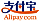Bookcover of Fixed Point Theorems in Fuzzy Metric Spaces
Booktitle:

# Fixed Point Theorems in Fuzzy Metric Spaces

LAP LAMBERT Academic Publishing (2016-03-30 )eligible for voucher
ISBN-13:

### 978-3-659-86660-9

ISBN-10:
3659866601
EAN:
9783659866609
Book language:
English
Blurb/Shorttext:
This book deals with a concise study of fixed point theorems in fuzzy metric spaces. This book mainly contains the authors' own research work in the area of fuzzy metric spaces. Fuzzy metric spaces have been an increasingly popular area of mathematical research in recent times, both in terms of theory and applications. But the availability of books in the area of fuzzy metric spaces is very rare. This book provides a good discussion on the development of both fuzzy and generalized fuzzy metric theory. The transition from fuzzy spaces to generalized fuzzy metric spaces has been presented systematically. Anybody interested in the theory or application of fuzzy metric spaces will find this book more than useful. The book is written in such a way that mathematical prerequisites are minimum. Since the main subject of study in this book is a generalization of the concept of usual fuzzy metric, so all the related results in fixed point have been incorporated in the book. This book may be used as a ready reference for an up to date account of results in the theory of fuzzy metric spaces at large.The concepts in this book have been explained with suitable examples whenever applicable.
Publishing house:
Website:
https://www.lap-publishing.com/
By (author) :
Sudipta Paul, Sanchita Chetia
Number of pages:
120
Published at:
2016-03-30
Stock:
Available
Category:
Mathematics
Price:
54.90 €
Keywords:
Fixed point theory, fuzzy metric space, Fuzzy Set, fuzzy contraction, fuzzy graph.### Categories